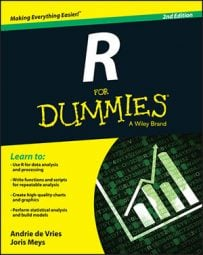##### R For DummiesIn addition to the mean and variation, you also can take a look at the quantiles in R. A quantile, or percentile, tells you how much of your data lies below a certain value. The 50 percent quantile, for example, is the same as the median. Again, R has some convenient functions to help you with looking at the quantiles.

## Calculating the range

The most-used quantiles are actually the 0 percent and 100 percent quantiles. You could just as easily call them the minimum and maximum, because that’s what they are. You can get both together using the range() function. This function conveniently gives you the range of the data. So, to know the range of mileages, you simply do:

```> range(cars\$mpg)
 10.4 33.9```

## Calculating the quartiles

The range still gives you only limited information. Often statisticians report the first and the third quartile together with the range and the median. These quartiles are, respectively, the 25 percent and 75 percent quantiles, which are the numbers for which one-fourth and three-fourths of the data is smaller. You get these numbers using the quantile() function, like this:

```> quantile(cars\$mpg)
0%  25%  50%  75%  100%
10.400 15.425 19.200 22.800 33.900```

The quartiles are not the same as the lower and upper hinge calculated in the five-number summary. The latter two are, respectively, the median of the lower and upper half of your data, and they differ slightly from the first and third quartiles. To get the five number statistics, you use the fivenum() function.

## Getting on speed with the quantile function

The quantile() function can give you any quantile you want. For that, you use the probs argument. You give the probs (or probabilities) as a fractional number. For the 20 percent quantile, for example, you use 0.20 as an argument for the value. This argument also takes a vector as a value, so you can, for example, get the 5 percent and 95 percent quantiles like this:

```> quantile(cars\$mpg, probs = c(0.05, 0.95))
5%  95%
11.995 31.300```

The default value for the probs argument is a vector representing the minimum (0), the first quartile (0.25), the median (0.5), the third quartile (0.75), and the maximum (1).

The argument na.rm allows you to remove all NA values before calculating the respective statistic. If you don’t do this, any vector containing NA will have NA as a result. This works identically to the na.rm argument of the sum() function.